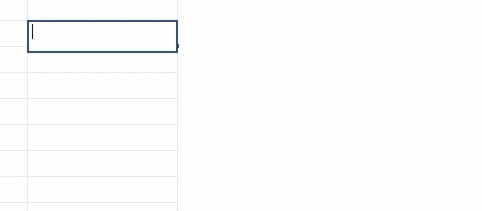# What would the formula be for.....

I need to sum or count the number of people in my sheet that are under 17 and over 65, based on who is checked.  Can I do this using a cross sheet formula so all my totals are together?  I have both DOB and age columns - can the formula be on the age column or do I need a value column to set age parameters?

• =countifs(age:age, "> 65") will give you the age of everyone greater than 65

=countifs(age:age, "<17") will givey you the age of everyone younger than 17.

You can use a reference from another sheet. Check out my animated gif to see how.• When you reference another sheet you will be able to set up a range from the sheet to pull into your new sheet. If you want the total number of people Over 65 and under 17 you can add the two countifs together. =countifs(age:age, "> 65")+countifs(age:age, "<17")

## Help Article Resources

Want to practice working with formulas directly in Smartsheet?

Check out the Formula Handbook template!